## Haar Function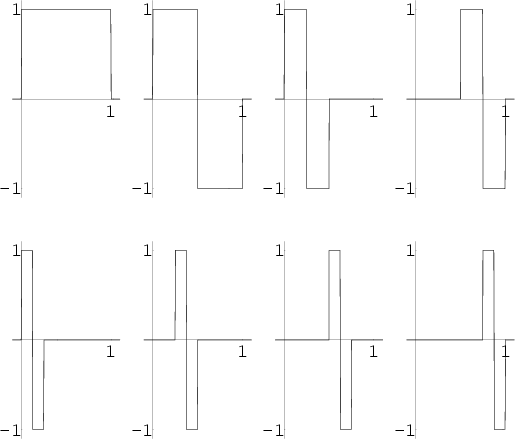Define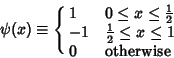(1)

and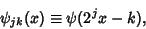(2)

where the Functions plotted above are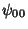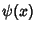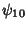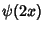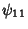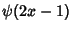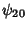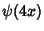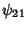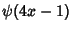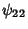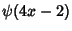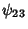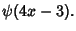Then a Function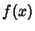can be written as a series expansion by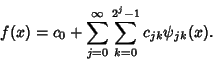(3)

The Functions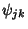and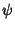are all Orthogonal in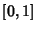, with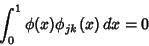(4)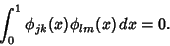(5)

These functions can be used to define Wavelets. Let a Function be defined onintervals, witha Power of 2. Then an arbitrary function can be considered as an-Vector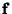, and the Coefficients in the expansion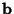can be determined by solving the Matrix equation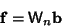(6)

for, where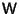is the Matrix ofbasis functions. For example,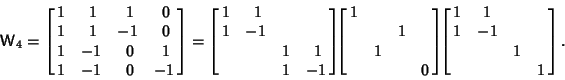(7)

The Wavelet Matrix can be computed in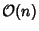steps, compared to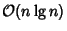for the Fourier Matrix.

Haar, A. Zur Theorie der orthogonalen Funktionensysteme.'' Math. Ann. 69, 331-371, 1910.
Strang, G. Wavelet Transforms Versus Fourier Transforms.'' Bull. Amer. Math. Soc. 28, 288-305, 1993.